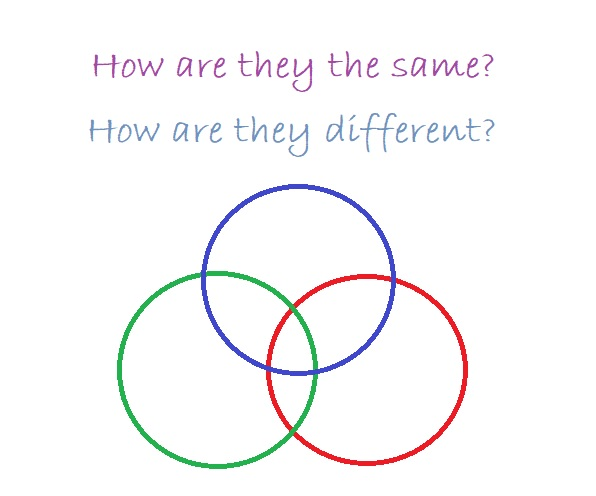# 1180 How Are They the Same? How Are They Different?

I liked the vintage buggies at Sosto Museum Village so much that I had to snap a picture and share them with you.

How are they the same? How are they different? When you answer those questions, you are doing mathematics!You could write the answers to those questions in a Venn Diagram. Then it would be more obvious that you are thinking mathematically!The number 1180 is like some other numbers but different than others. Here are some facts about it:

• 1180 is a composite number.
• Prime factorization: 1180 = 2 × 2 × 5 × 59, which can be written 1180 = 2² × 5 × 59
• The exponents in the prime factorization are 2, 1, and 1. Adding one to each and multiplying we get (2 + 1)(1 + 1)(1 + 1) = 3 × 2 × 2 = 12. Therefore 1180 has exactly 12 factors.
• Factors of 1180: 1, 2, 4, 5, 10, 20, 59, 118, 236, 295, 590, 1180
• Factor pairs: 1180 = 1 × 1180, 2 × 590, 4 × 295, 5 × 236, 10 × 118, or 20 × 59
• Taking the factor pair with the largest square number factor, we get √1180 = (√4)(√295) = 2√295 ≈ 34.351131180 is the hypotenuse of a Pythagorean triple:
708-944-1180 which is (3-4-5) times 236

1180 is the sum of two consecutive prime numbers: 587 + 593 = 1180

1180 is palindrome 1551 in BASE 9 because 1(9³) + 5(9²) + 5(9) + 1(1) = 1180